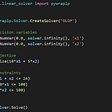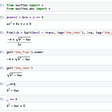# The theorem

`x = a1 (mod n1)...x = ak (mod nk)`

# The proof

`x = a1 (mod n1)x = a2 (mod n2)`
`y = a1 * q * n2 + a2 * p * n1  (mod N)`
`y = sum(ai * (N / ni) * invmod(N / ni, ni)`
`z = y (mod N)`

# Algorithm 1: Gauss algorithm

`def ChineseRemainderGauss(n, N, a):    result = 0    for i in range(len(n)):        ai = a[i]        ni = n[i]        bi = N // ni        result += ai * bi * invmod(bi, ni)    return result % N`

# Algorithm 2: Euclid

`x = sum(ai * b * (N / ni))  for i=1...k`
`def ChineseRemainderEuclid(n, N, a):    result = 0    for i in range(len(n)):        ai = a[i]        ni = n[i]        _, _, si = ExtendedEuclid(ni, N // ni)        result += ai * si * (N // ni)    return LeastPositiveEquivalent(result, N)`

# The extended Euclidean algorithm

`a * x + b * y = gcd(a, b)`
`x = qy + r`
`x = q1 * y + r1q1 = q2 * r + r2...`
`gcd(102, 38)102 = 2*38 + 2638 = 1*26 + 1226 = 2*12 + 212 = 6*2 + 0`
`2 = 26 - 2*122 = 26 - 2*(38 - 1*26) = 26 - 2*(38 - 1*(102 - 2*38))2 = 3*102 - 8*38`
`def ExtendedEuclid(x, y):    x0, x1, y0, y1 = 1, 0, 0, 1    while y > 0:        q, x, y = math.floor(x / y), y, x % y        x0, x1 = x1, x0 - q * x1        y0, y1 = y1, y0 - q * y1    return a, x0, y0  # gcd and the two coefficients`

# The modular inverse

`17*x = 1 (mod 43)`
`Forward:43 = 17*2 + 917 = 9*1 + 89 = 8*1 + 1  # <-- my GCD is here, so it is 1Backward:1 = 9 - 8*18 = 17 - 9*19 = 43 - 17*2Replacing the first "backward" equation with everything else:1 = 43 - 17*2 - 17 + 43 - 17*2 = 2*43 - 17*5`
`2*43 - 5*17 = 1 (mod 43)`
`-5*17 = 1 (mod 43)`
`def invmod(a, m):	g, x, y = ExtendedEuclid(a, m)	if g != 1:	    raise ValueError('modular inverse does not exist')	else:	    return x % m`

# References

--

--

--

## More from Astarte Kraus

Random lady whose eyes sparkle for science, and who loves writing. I plan to write about a large variety of interesting science topics, one bit at a time.

Love podcasts or audiobooks? Learn on the go with our new app.

## How to run Headless Chrome in scale## Deployment with Kubernetes## Bitrise- Flavor Wise Workflow for Flutter Integration Part-2## Hadoop Job Roles & Benefits — Latest updated (2020)## Introducing REN, BAO, PERP, LINA & BADGER futures trading on FMX## The First Quarter Contributors Selection## Astarte Kraus

Random lady whose eyes sparkle for science, and who loves writing. I plan to write about a large variety of interesting science topics, one bit at a time.

## 4 Ways to Solve Linear Programming in Python## You’ll Never Look at the Bias-Variance Tradeoff the Same Way Again!## Calculus — Curves## Faster math typesetting in Jupyter and Python (Part 3)